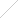Mathematica Link for ExcelFeaturesWhat's New in Version 3Who's It For?ExamplesUser's Manual and Reference GuideBuy Online###Bringing the Power of Mathematica to ExcelThis one's easy: You own Mathematica. You own Microsoft Excel. You need Mathematica Link for Excel! Have the best of both worlds at your fingertips: all the power of Mathematica and all the convenience of Excel. Mathematica Link for Excel makes working with both programs seamless.

Forget about creating cumbersome temporary data files. With Mathematica Link for Excel, you can easily copy and paste data between Mathematica and Excel or experience unparalleled side-by-side use of both programs while you programmatically read and write data from Mathematica notebooks to open Excel workbooks. You can even convert Mathematica notebooks into Excel macros in just a few simple steps.

For Excel users, Mathematica Link for Excel adds over a thousand functions and options to Excel and lets you interactively explore them using the Mathematica function wizard. Excel formulas can suddenly perform symbolic computations, find complex roots, run statistical analysis and much more. Even displaying Mathematica's legendary graphics and typeset equations becomes as easy as entering an Excel formula. Go ahead. From a quick histogram to a 3D vector plot, Mathematica Link for Excel lets you visualize your data in ways not possible using Excel alone.

Key Features

Mathematica Link for Excel is a fully bidirectional link that can be used to:

• Copy and paste data between Mathematica and Excel
• Write code in Mathematica that interacts with and automates Excel
• Develop customized Excel import and export routines in Mathematica
• Call Mathematica code as if it were an Excel macro
• Create spreadsheet formulas that call Mathematica from within Excel
• Display Mathematica graphics and typeset expressions in Excel

Mathematica Link for Excel 3.7.1 requires Mathematica 8 or greater and Microsoft Excel 2010 or newer. It is available for Windows 7, 8, 8.1 or 10. Earlier software and operating systems may be supported with a few limitations. The ExcelLink package for Mathematica is compatible with both 32-bit and 64-bit versions of Mathematica and Excel. The Mathematica Link add-in for Excel is compatible only with the 32-bit version of Excel.